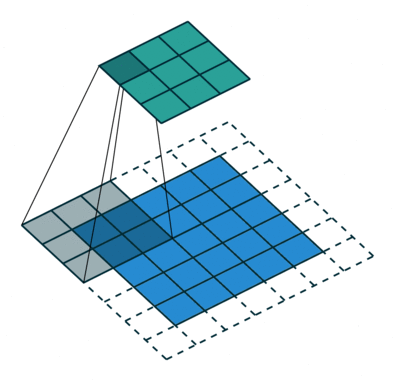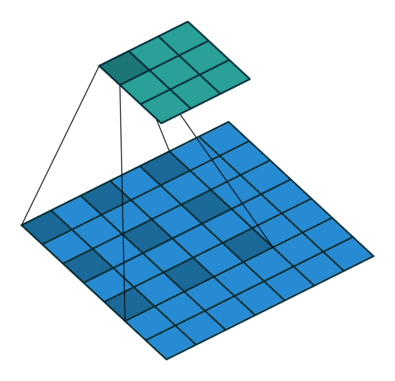MathWorks 官網有對 Semantic Segmantation 做了一個基礎的介紹# 1. Introduction

• Volume representation 最能夠「完整地」描述一張圖片，直觀上也是最容易實做的，但是礙於硬體資源（記憶體、容量等），所以不大可行。
• Multi-view representation 雖然不是最真實的描述方式，但能夠透過事先訓練好的 2D 模型來加以實現。
• Point clouds 不但資訊量較小，而且較為彈性，但 point clouds 與神經網路的應用還未被深入挖掘，這也是此篇 paper 誕生的原因。

• A pointwise convolution operator that can output features at each point in a point cloud;
• Two pointwise convolutional neural networks for semantic scene segmentation and object recognition.# 2. Related Works

## 2.1. Shape descriptors

Hand-crafted 的 shape descriptors 在深度學習誕生以前，時常被運用在各種不同的 computer vision 應用上，直到深度學習的出現，傳統方法的眼淚也滴下來了。

## 2.2. Object recognition

ImageNet 就是一個著名的大型 RGB 3 維 image dataset，CNNs 能夠從中成功地學習到 image descriptors 並大勝傳統方法。

PointNet 是第一個能夠處理 point cloud data 的網路架構，他能夠學習到順序不變性（order-invariance）的函式。

Stanford 提出的 PointNet 雖然效能很猛，但網路複雜，這篇 paper 提出了相對簡單的 pointwise convolution，且能達到和 PointNet、PointCNN 等相仿的準確度。

## 2.3. Semantic segmentation

RGB-D 圖片其實是兩張圖片：

• 普通的 RGB 3 維圖片
• Depth 圖片（類似灰階圖片，只是每個像素值是距離物體的實際距離）

SegNet 就在此 dataset 得到不錯的效果，他使用的方法為：

• encoder-decoder
• dilation filter

McCormac 則能透過 2D 預測 3D domain，但這些預測並無法被直接應用在 3D domain。

SSCNet 應用 CNN 在 3D volume representation 去分類每個像素。

# 3. Pointwise Convolution

Point clouds 能夠被 RGB-D reconstruction 和 CAD modeling 的特性，因此也是一個不錯的表示法，PointNet 就是基於此產生的，但將 point cloud 餵給神經網路是不自然的，因為傳統的 convolution operators 只設計給 grid 和 volumes。

## Convolution

• 以 point cloud 中每一個點為中心
• 有一個 size 或 radius value（可被調整）$$x_i^\ell = \sum_k w_k \frac{1}{|\Omega_i(k)|} \sum_{p_j \in \Omega_i(k)} x_j^{\ell - 1}, \tag{1}$$

• $k$：所有 sub-domains
• $\Omega_i(k)$：當 kernel 以 point $i$ 為中心時，第 $k$-th sub-domain
• $p_i$：point $i$ 的座標
• $|\cdot|$：計算所有 sub-domain 裡的 points 數量
• $w_k$：$k$-th sub-domain 的 kernel weight
• $x_i$ 和 $x_j$：point $i$ 和 $j$ 的值
• $\ell - 1$ 和 $\ell$：input 和 output index

$$\frac{\partial L}{\partial x_j^{\ell - 1}} = \sum_{i \in \Omega_j} \frac{\partial L}{\partial x_i^\ell} \frac{\partial x_i^\ell}{\partial x_j^{\ell - 1}}, \tag{2}$$

$$\frac{\partial x_i^\ell}{\partial x_j^{\ell - 1}} = \sum_k w_k \frac{1}{|\Omega_i(k)|} \sum_{p_j \in \Omega_i(k)} 1 \tag{3}$$

$$\frac{\partial L}{\partial w_k} = \sum_i \frac{\partial L}{\partial x_i^\ell} \frac{\partial x_i^\ell}{\partial w_k} \tag{4}$$

$$\frac{\partial x_i^\ell}{\partial x_k} = \frac{1}{|\Omega_i(k)|} \sum_{p_j \in \Omega_i(k)} x_j^{\ell - 1} \tag{5}$$

1. 不再需要處理 downsampling 和 upsampling
2. 鄰居 query 的加速結構只需要建構一次

## Point order## À-trous convolution

GIF 來源# 4. Evaluations

## Semantic segmantationTable 1: Comparison of scene segmentation on S3DIS dataset Table 2: Per-class accuracy of semantic segmentation on S3DIS dataset Table 3: Per-class accuracy of semantic segmentation on SceneNN datasetSemantic segmentation on the S3DIS dataset Semantic segmentation on SceneNN dataset

## Object recognitionTable 4: Comparison of performance of network architectures using 3D object representations on the ModelNet40 dataset Table 5: Comparison of object recognition accuracy on the ObjectNN dataset

1794 Words

2018-09-27 15:04 -0400